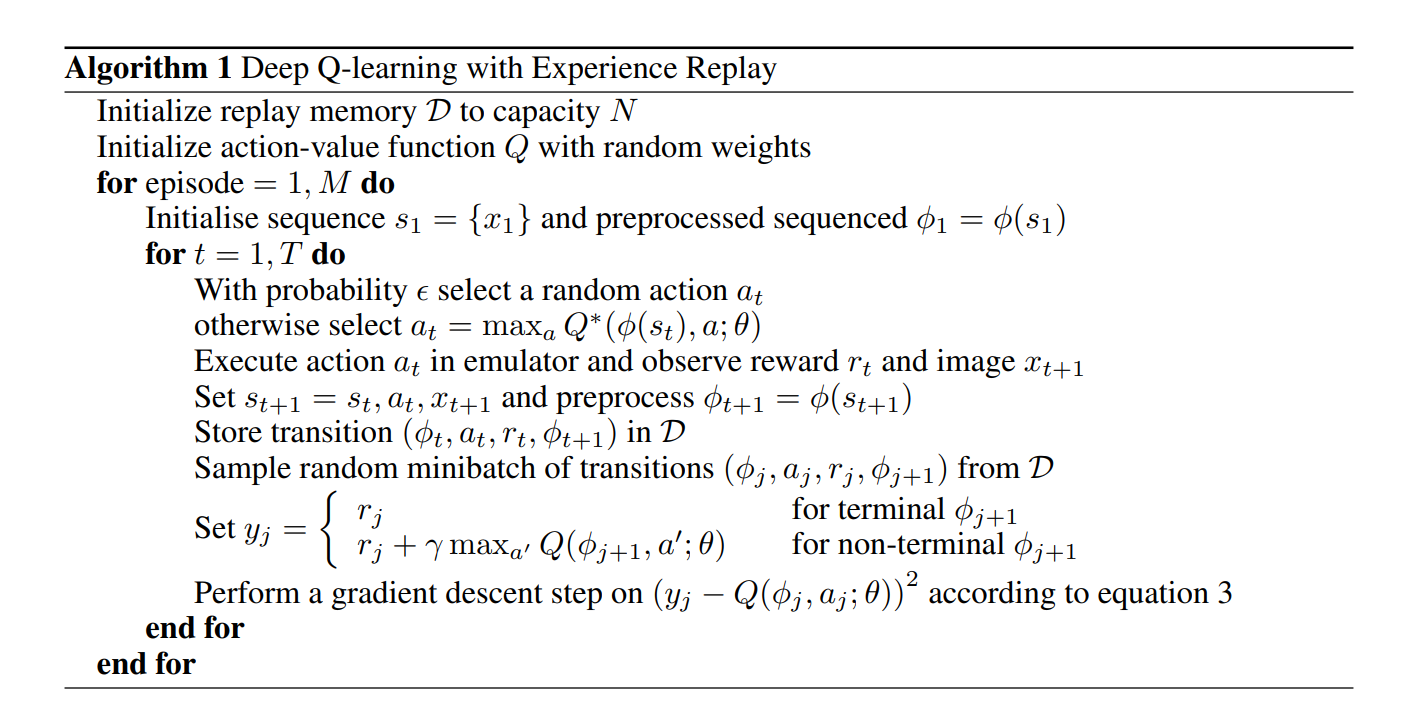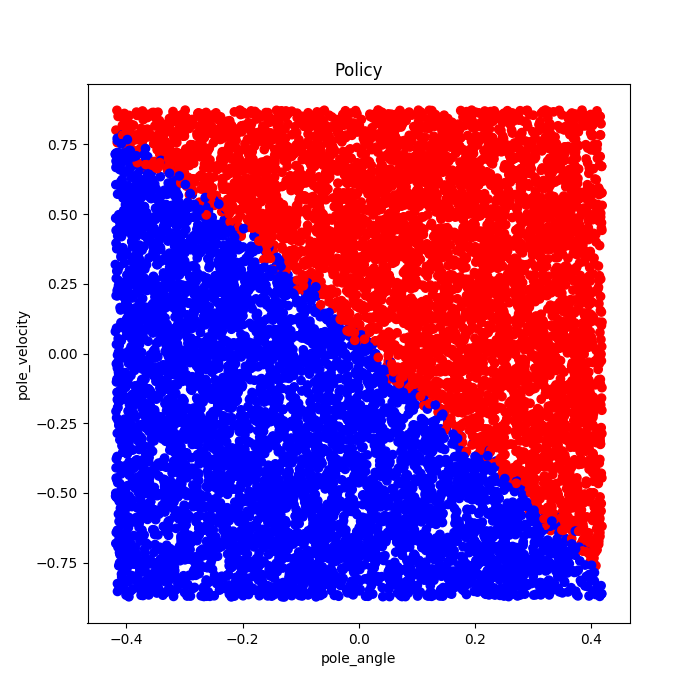0%

## Introduction

There are two kinds of methods in reinforcement learning, tabular methods and approximate methods. The purpose of RL is to get an optimal policy, which tells you to choose what action A when you at state S. If the state and aciton spaces are small enough, value fucntion can be represented as arrays, or tables. The problem with large state space is not just the memory needed for large tables, but the time and data needed to fill them accurately. If the state and aciton spaces are too large, due to the limitions of time and data, value functions need to be approximated with limited computational resources. In this case, out goal instead is to find a good enough approximate solution compared to optimal solution. This post is some reading notes for Playing Atari with Deep Reinforcement learning which published by DeepMind(2013). Instead playing Atari games, I play CartPole games whose simulation environment is provided by Openai Gym. I implement the algorithm proposed by the DeepMind which known as DQN to solve CartPole. Besides, a discrete-q-learing method is discussed compared to DQN.

CartPole is a classic control task which has infinite state space and finite action space. It is difficult to solve the problem use tabular methods. A neural network can solve this problem to learn successful control polcies. The network is trained with a variant of the Q-learning algorithm, with stochastic gradient descent to update the weights. To alleviate the problems of correlated data and non-stationary distributions, we use an experience replay mechanism which randomly samples previous transitions, and thereby smooths the training distribution over many past behaviors.

## Background

We consider tasks in which an agent interacts with an environment $\epsilon$, in this case the openai gym emulator, in a sequence of actions, observations and rewards. At each time-step the agent selects an action $a_t$ from the set of legal game actions, $A=${0, 1}. The action is passed to the emulator and it returns a list of next_state, reward, done.

We consider sequences of actions and observations, $s_t=x_1, a_1, x_2,…, a_{t-1},x_t$ and learn game strategies that depend opon these sequences. All sequences in the emulator are assumed to terminate in a finite number of time-steps. This formalism gives rise to a large but finite Markov decision process(MDP) in which each sequence is a distinct state. As a result, we can apply standard reinforcement learning methods for MDPs, simply by using the complete sequence $s_t$ as the state representation at time t.

The goal of the agent is to interact with the agent by selecting actions in a way that maximize future rewards. We some following definitons:

• We make the standard assumption that future rewards are discounted by a factor of $\gamma$ per time-step.
• The future discounted return at time $t$ as $R_t=\Sigma_{t’=t}^{T}\gamma^{t’-t}r_{t’}$, where $T$ is the time step at which the game terminates.
• Optimal action-value function $Q^*(s, a)$ as the maximum expected return achievable by following any strategy, after seeing some sequence $s$ and then taking some action $a$, $Q^*(s, a)=max_\pi E[R _ t|s _ t=s,a _ t=a,\pi]$, where $\pi$ is a policy mapping sequences to actions (or distributions over actions).

The optimal action-value function obeys an important identity known as the Bellman equation. This is based on the following intuition: if the optimal value $Q^*(s’, a’)$ of the sequence $s’$ at the next time-step was known for all possible actions $a’$, then the optimal strategy is to select the action $a’$ maximizing the expected value of $r+\gamma Q^*(s’, a’)$

$$Q^*(s, a)=E_{s’ \in \epsilon}[r+\gamma max_{a’}Q^*(s’, a’|s, a)]$$

Such value iteration algorithms converge to the optimal action value function, $Q_i \rightarrow Q^*(s, a)$ as $i \rightarrow \infty$

In practice, this basic approach is totally impractical, because the action-value function is estimated separately for each sequence, without any generalisation. Instead, it is common to use a function approximator to estimate the action-value function, $Q(s, a; \theta)=Q^*(s, a)$. In the reinforcement learning community this is typically a linear function approximator, but sometimes a non-linear function aproximator is used instead, such as a neural network. We refer to a neural network function approximator with weights $\theta$ as a Q-network. A Q-network can be trained by minimizing a sequence of loss functions $L_i(\theta_i)$ that changes at each iteration i.

$$L_i(\theta_i)=E_{s,a \in \rho(\cdot)}[(y _ i-Q(s,a;\theta _ i))^2]$$

where $y_i=E_{s’ \in \epsilon}[r+\gamma max_{a’}Q(s’, a’;\theta_{i-1}|s, a)]$ is the target for iteration $i$ and $\rho(s, a)$ is a probability distribution over sequences $s$ and aciton $a$ that we refer to as the behaviour distribution.

## Deep Reinforcement Learning

### DQN pseudocode## Result

After training for 1000 episodes, we get the following policy:### How to compute TD Loss

In contrast to TD-Gammon and similar online approaches, we utilize a technique known as experience replay where we store the agent’s experiences at each time-step, $e_t=(s_t, a_t, r_t, _{t+1})$ in a data-set $D=e_1, e_2, … ,e_N$, pooled over many episodes into a replay memory.

Since we have known the pair $(s_t, a_t)$, we can get the q_value = q_values.gather(1, action.unsqueeze(1)).squeeze(1). Then, we know next_state, compute next_q_value. Choose $a’$ which maximize the next_q_value.

## Reference

 Mnih, Volodymyr, et al. “Playing atari with deep reinforcement learning.” arXiv preprint arXiv:1312.5602 (2013).

 https://stackoverflow.com/questions/50999977/what-does-the-gather-function-do-in-pytorch-in-layman-terms

 https://stackoverflow.com/questions/57237352/what-does-unsqueeze-do-in-pytorch

 https://gist.github.com/ts1829/62b9ae7687d79279b9381bb6808bf6ab#file-mountain-car-v0-q-learning-modified-reward-ipynb

If you like my blog, please donate for me.